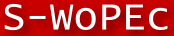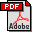Scandinavian Working Papers in Economics

# No 28/2014: Serially Correlated Measurement Errors in Time Series Regression: The Potential of Instrumental Variable Estimators

Erik Biørn ()
Erik Biørn: Dept. of Economics, University of Oslo, Postal: Department of Economics, University of Oslo, P.O Box 1095 Blindern, N-0317 Oslo, Norway

Abstract: The measurement error problem in linear time series regression, with focus on the impact of error memory, modeled as finite-order MA processes, is considered. Three prototype models, two bivariate and one univariate ARMA, and ways of handling the problem by using instrumental variables (IVs) are discussed as examples. One has a bivariate regression equation that is static, although with dynamics, entering via the memory of its latent variables. The examples illustrate how 'structural dynamics' interacting with measurement error memory create bias in Ordinary Least Squares (OLS) and illustrate the potential of IV estimation procedures. Supplementary Monte Carlo simulations are provided for two of the example models.

JEL-codes: C22; C26; C32; C36; C52; C53

16 pages, December 30, 2014

Full text files

`memo-28-2014.pdf`